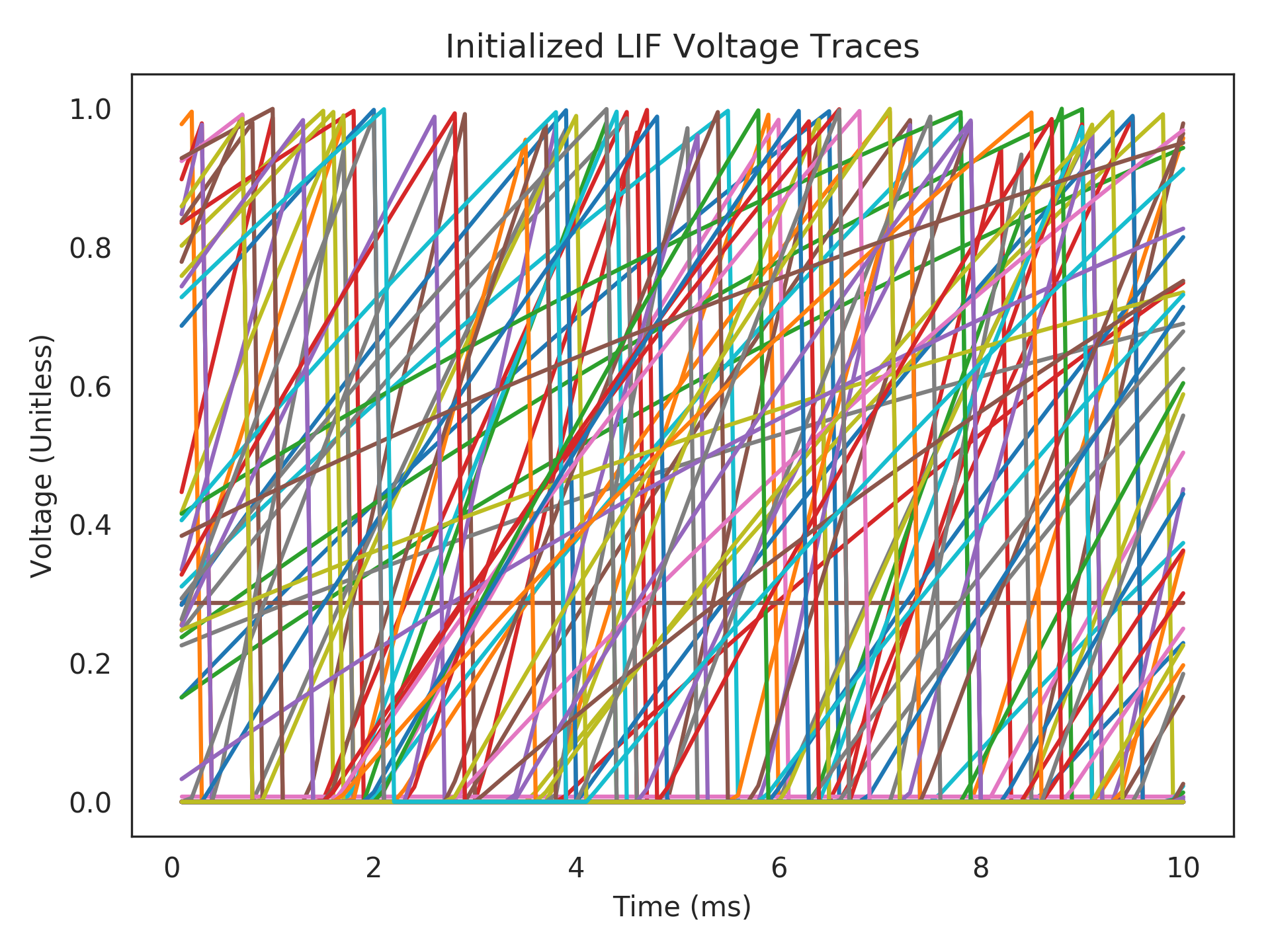# nengolib.neurons.init_lif¶

nengolib.neurons.init_lif(sim, ens, x0=None, rng=None)[source]

Initialize an ensemble of LIF Neurons to represent x0.

Must be called from within a simulator context block, and before the simulation (see example below).

Parameters: sim : nengo.Simulator The created simulator, from whose context the call is within. ens : nengo.Ensemble The ensemble of LIF neurons to be initialized. x0 : (d,) array_like, optional A d-dimensional state-vector that the ensemble should be initialized to represent, where d = ens.dimensions. Defaults to the zero vector. rng : numpy.random.RandomState or None, optional Random number generator state. v : (n,) np.array Array of initialized voltages, where n = ens.n_neurons. r : (n,) np.array Array of initialized refractory times, where n = ens.n_neurons.

Notes

This will not initialize the synapses.

Examples

>>> import nengo
>>> from nengolib import Network
>>> from nengolib.neurons import init_lif
>>>
>>> with Network() as model:
>>>      u = nengo.Node(0)
>>>      x = nengo.Ensemble(100, 1)
>>>      nengo.Connection(u, x)
>>>      p_v = nengo.Probe(x.neurons, 'voltage')
>>>
>>> with nengo.Simulator(model, dt=1e-4) as sim:
>>>      init_lif(sim, x)
>>>      sim.run(0.01)
>>>
>>> import matplotlib.pyplot as plt
>>> plt.title("Initialized LIF Voltage Traces")
>>> plt.plot(1e3 * sim.trange(), sim.data[p_v])
>>> plt.xlabel("Time (ms)")
>>> plt.ylabel("Voltage (Unitless)")
>>> plt.show()